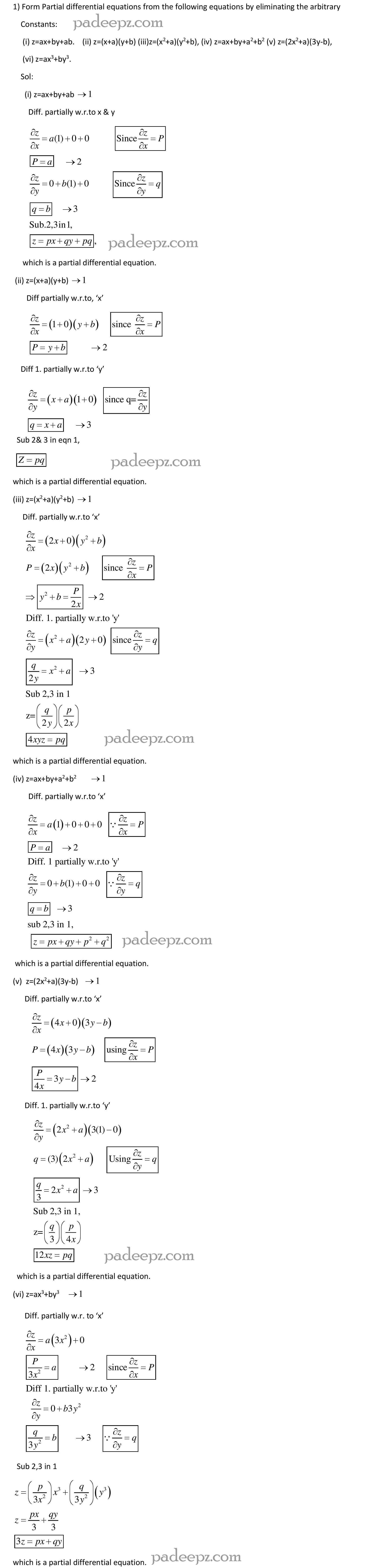# MA8353 Question Bank Transforms and Partial Differential Equations

MA8353 Question Bank Transforms and Partial Differential Equations Regulation 2017 Anna University free download. Transforms and Partial Differential Equations Question Bank MA8353 pdf free download Question Paper.

## Sample MA8353 Question Bank Transforms and Partial Differential Equations:

1. Form the partial differential equation by eliminating a and b from z  a(x  y) 

2. Form the partial differential equation by eliminating a and b from ( )( ) 2 2 2 2 z  x  a y  b MA8353 Question Bank Transforms and Partial Differential Equations Question Paper

3. Form the partial differential equation by eliminating from z  a x  ay  b 2 2

4. Form the partial differential equation by eliminating a and b from 2 2 z  (x  a)  (y  b)

5. Find the singular integral of the PDE 2 2 z  px  qy  p  q

6. Solve ( 2 ‘ ‘ ) 0 2 2 D  DDD z 

7. Solve ( 2 ‘ ) 0 3 D  DD z 

8. Solve ( ‘ ) 0 3 D D  MA8353 Question Bank Transforms and Partial Differential Equations Question Paper

9. Solve (D  2D’ )(D  2D’1)z  0

10. Solve (4 4 ‘ ‘ ) 0 2 2 D  DDD z 

11. Find the general solution of 0

12. Find the PI of (D  3DD’2D’ )z  x  y 2 2

13. Find the PI of x y D DD D z e  (  2 ‘ ‘ )  2 2

14. Solve y MA8353 Question Bank Transforms and Partial Differential Equations

1. Form the partial differential equation by eliminating a and b from 2 2 2 2 (x  a)  (y  b)  z  c

2. Form the partial differential equation by eliminating arbitrary functions from
z  xf (2x  y)  g(2x  y)

3. Form the partial differential equation by eliminating arbitrary functions f and  from z  f (x  ct) (x  ct) MA8353 Question Bank Transforms and Partial Differential Equations Question Paper

4. Form the partial differential equation by eliminating arbitrary functions g from
( , ) 0 2 2 2 g x  y  z xyz 

5. Solve (1 ) (1 ) 2 p  q  q  z

6. Solve p(1 q)  qz

7. Solve pq  p  q  0

8. Solve 9( ) 4 2 2 p z  q 

9. Find the complete integral of 2 2 2 2 2 x p  y q  z

10. Solve 2 2 z  px  qy  p q and find the complete and singular solutions

### Padeepz E-Learning Materials MA8353 Transforms and Partial Differential Equations

Padeepz E-Learning Materials for MA8353 Transforms and Partial Differential Equations we have provided the sample materials in this page.  If you like the sample and want to buy the full subject the procedure is also provided in this page.Hi Padeepz.com is a place where we make Engineering Students to understand their subjects in an easy and in a effective way. Which leads to greater knowledge in the subject and Provide plenty of time spending in innovative ideas.

MA8353 Question Bank Transforms and Partial Differential Equations

 Subject name Transforms and Partial Differential Equations Semester 3 Subject Code MA8353 Regulation 2017 regulation

MA8353 Question Paper Transforms and Partial Differential Equations

MA8353 Important questions Transforms and Partial Differential Equations

MA8353 Syllabus Transforms and Partial Differential Equations

MA8353 Notes Transforms and Partial Differential Equations